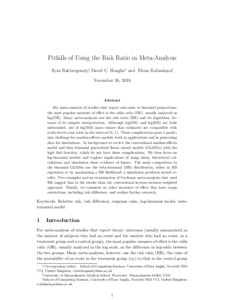# Pitfalls of using the risk ratio in meta‐analysis

Bakbergenuly, Ilyas, Hoaglin, David and Kulinskaya, Elena (2019) Pitfalls of using the risk ratio in meta‐analysis. Research Synthesis Methods, 10 (3). pp. 398-419. ISSN 1759-2879Preview PDF (RR_paper_26Nov18) - Accepted Version Download (985kB) | Preview

## Abstract

For meta-analysis of studies that report outcomes as binomial proportions, the most popular measure of effect is the odds ratio (OR), usually analyzed as log(OR). Many meta-analyses use the risk ratio (RR) and its logarithm, because of its simpler interpretation. Although log(OR) and log(RR) are both unbounded, use of log(RR) must ensure that estimates are compatible with study-level event rates in the interval (0, 1). These complications pose a particular challenge for random-effects models, both in applications and in generating data for simulations. As background we review the conventional random-effects model and then binomial generalized linear mixed models (GLMMs) with the logit link function, which do not have these complications. We then focus on log-binomial models and explore implications of using them; theoretical calculations and simulation show evidence of biases. The main competitors to the binomial GLMMs use the beta-binomial (BB) distribution, either in BB regression or by maximizing a BB likelihood; a simulation produces mixed results. Two examples and an examination of Cochrane meta-analyses that used RR suggest bias in the results from the conventional inverse-variance-weighted approach. Finally, we comment on other measures of effect that have range restrictions, including risk difference, and outline further research.

Item Type: Article relative risk, risk difference, response ratio, log-binomial model, beta-binomial model Faculty of Science > School of Computing Sciences LivePure Connector 08 Mar 2019 09:30 22 Oct 2022 04:32 https://ueaeprints.uea.ac.uk/id/eprint/70184 10.1002/jrsm.1347View Item